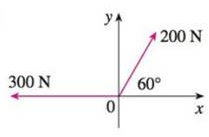Chapter 12.2, Problem 32E

Chapter
Section
Textbook Problem

# Find the magnitude of the resultant force and the angle it makes with the positive x-axis.33.To determine

Solution: The magnitude of resultant force and angle with positive x-axis are 28.7lb_ and 12.4°_ respectively.

Explanation

Given:

The magnitude of first vector as 20 lb and a angle with positive x-axis as 45° and the magnitude of second vector as 16 lb and angle with positive x-axis as 30° .

Formula:

Write the expression for vector in terms of horizontal and vertical components.

F=|F|cosθi+|F|sinθj (1)

Here,

|F| is magnitude of vector F.

Write the expression for resultant vector of two vectors F1 and F2 (F) .

F=F1+F2 (2)

Write the expression for magnitude of vector F1i+F2j .

|F|=F12+F22 (3)

Write the expression for tanθ of a vector F.

tanθ=F2F1 (4)

Substitute 20 lb for |F| , and 45° for θ in equation (1),

F1=(20lb)cos(45°)i+(20lb)sin(45°)j=(20lb)(12)i+(20lb)(12)j=102i+102jlb

Substitute 16 lb for |F| , and 30° for θ in equation (1),

F2=(16lb)cos(30°)i+(16lb)sin(30°)j=(16lb)(32)i+(16lb)(12)j=83

### Still sussing out bartleby?

Check out a sample textbook solution.

See a sample solution

#### The Solution to Your Study Problems

Bartleby provides explanations to thousands of textbook problems written by our experts, many with advanced degrees!

Get Started